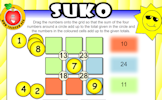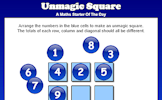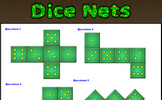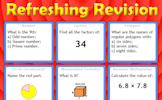## Year 11 Scheme of Learning

### Term 1 starting in week 1 :: Estimated time: 2 weeks

• Equations of lines parallel to the axis (review)
• Plot straight line graphs (review)
• Interpret $$y = mx + c$$ (review)
• Find the equation of a straight line from a graph (review)
• Equation of a straight-line graph given one point and gradient
• Equation of a straight-line graph given two points
• Determine whether a point is on a line
• Solve linear simultaneous equations graphically (review)

For higher-attaining pupils:

• Recognise when straight lines are perpendicular
• Find the equations of perpendicular lines

This page should remember your ticks from one visit to the next for a period of time. It does this by using Local Storage so the information is saved only on the computer you are working on right now.

## Lesson Starters

Here are some suggestions for whole-class, projectable resources which can be used at the beginnings of each lesson in this block.

### 1st Lesson#### Suko

Interactive number-based logic puzzle similar to those featuring in The Times and Telegraph newspapers.

### 2nd Lesson#### Stair Perimeter

Use the information implied in the diagram to calculate the perimeter of this shape.

### 3rd Lesson#### Unmagic Square

Arrange the numbers 1 to 9 in a 3 by 3 grid so that none of the line totals are the same.

### 4th Lesson#### Anagrams

Can you un-jumble these mathematical words?

### 5th Lesson#### Dice Nets

Determine whether the given nets would fold to produce a dice.

### 6th Lesson#### Refreshing Revision

It is called Refreshing Revision because every time you refresh the page you get different revision questions.

Some of the Starters above are to reinforce concepts learnt, others are to introduce new ideas while others are on unrelated topics designed for retrieval practice or and opportunity to develop problem-solving skills.

White Rose ResourcesEnd of block assessments provide a quick progress check at the end of each block of learning to make sure students have understood the content covered. This Scheme of Learning was produced by White Rose Maths and is used here with permission granted on 30th June 2021.For All: